# A2 gravitational field KYUEM Physics

10 Oct 2010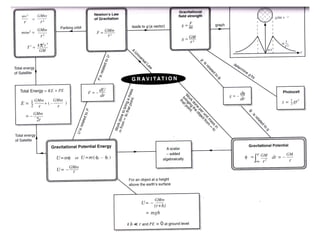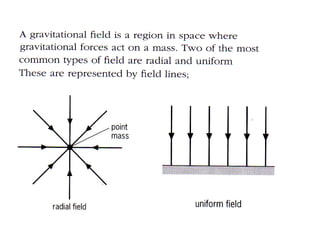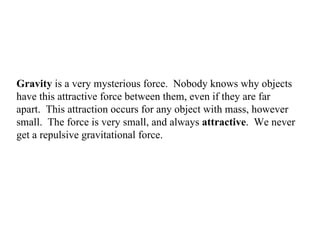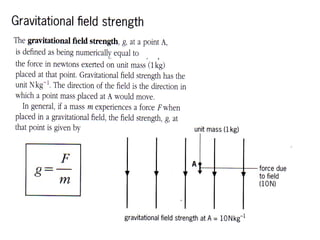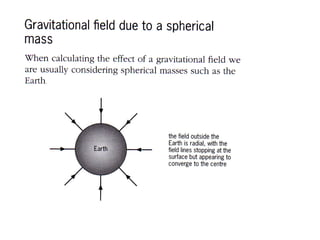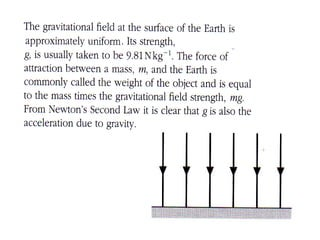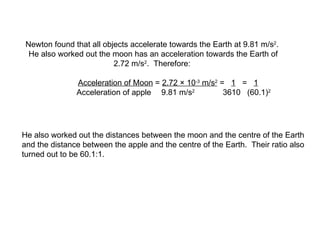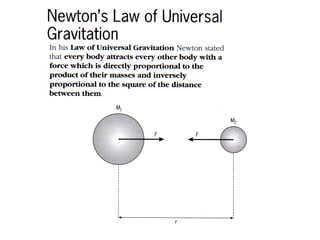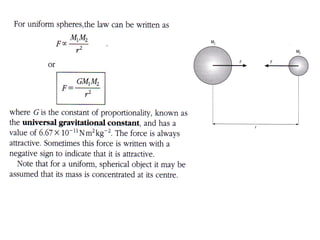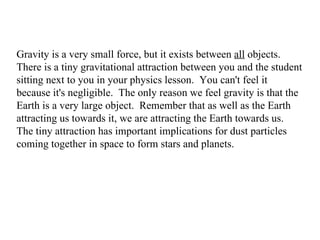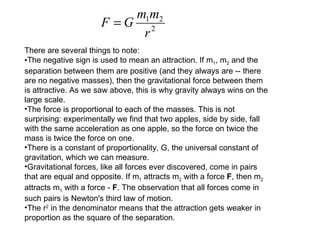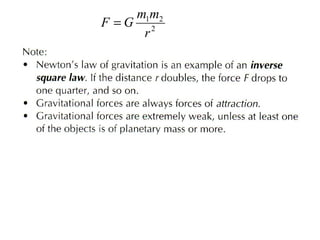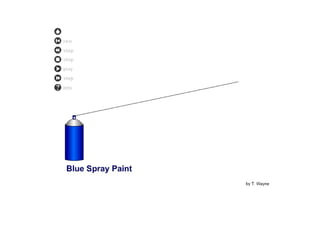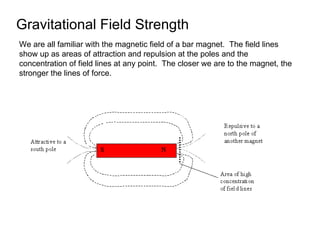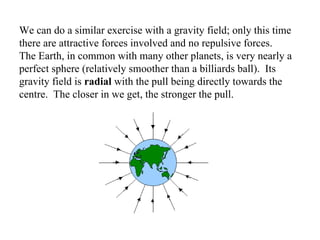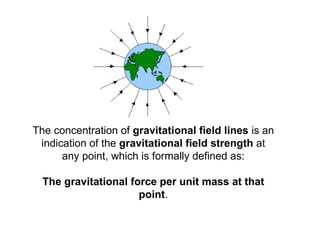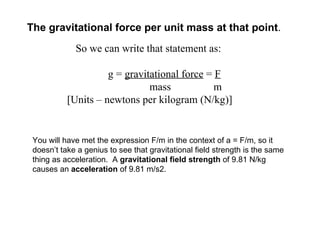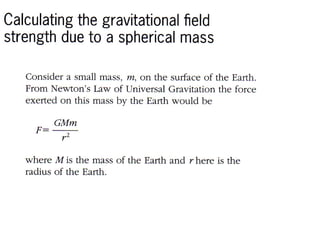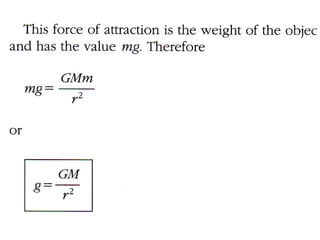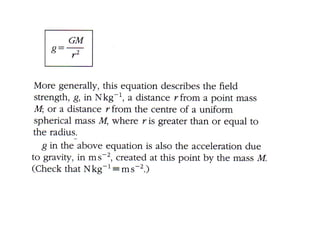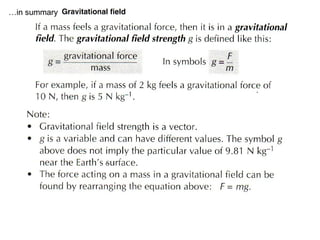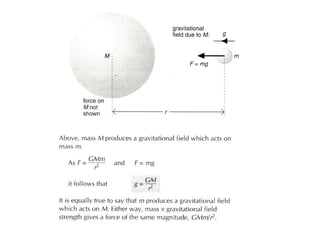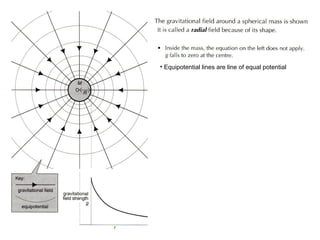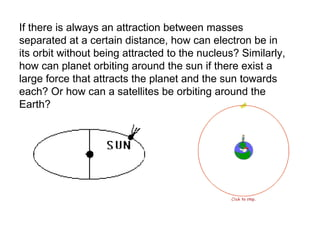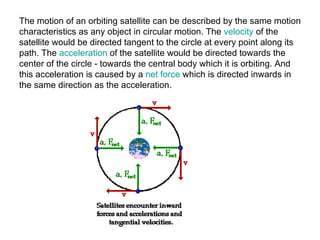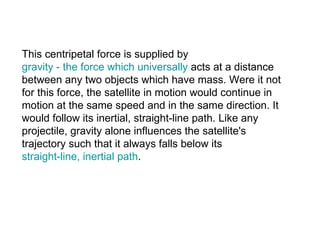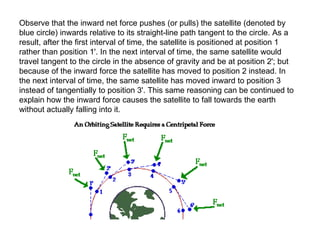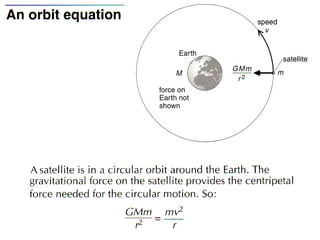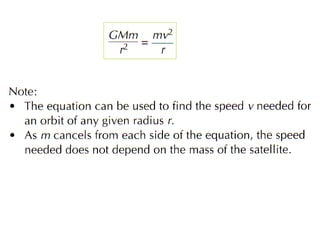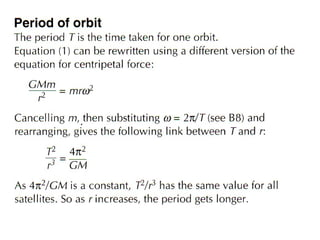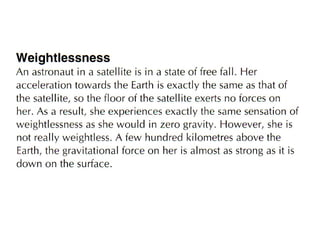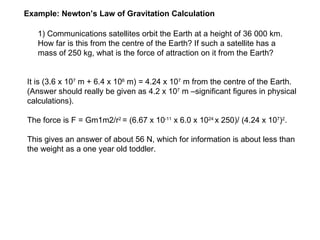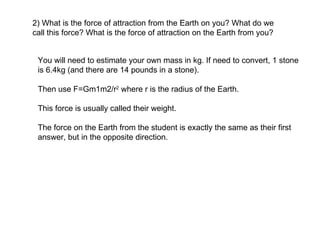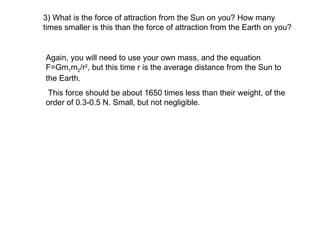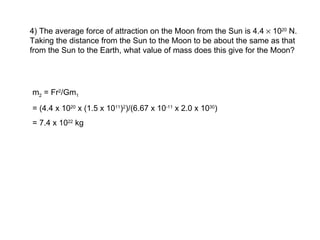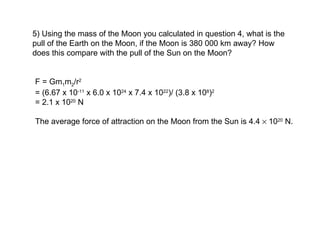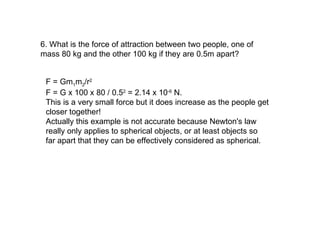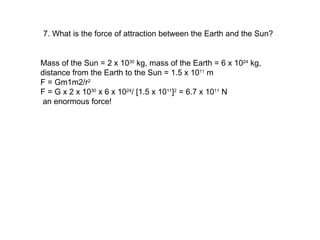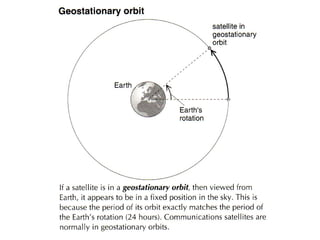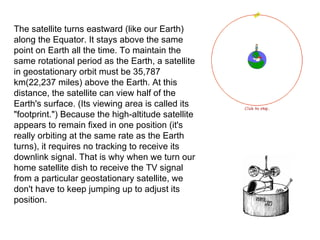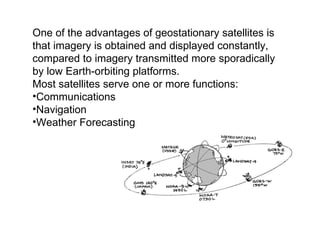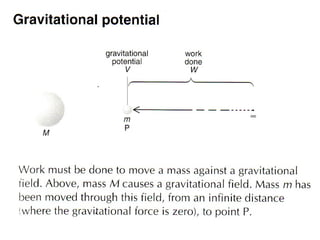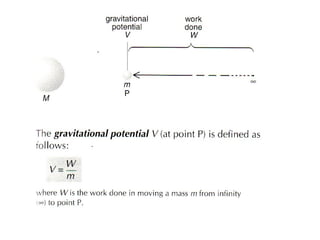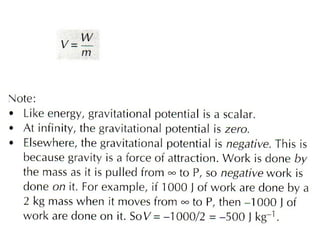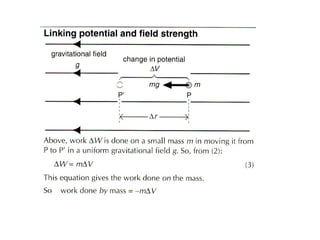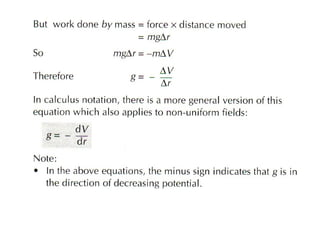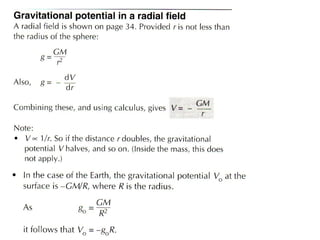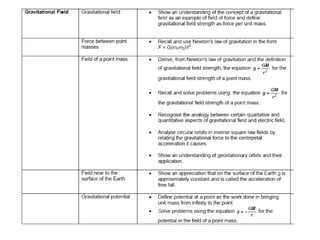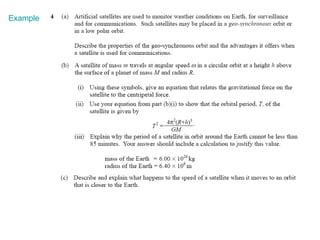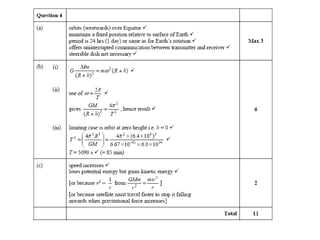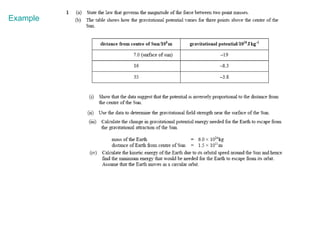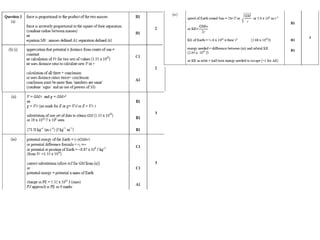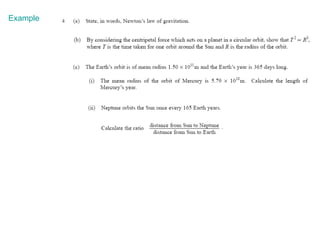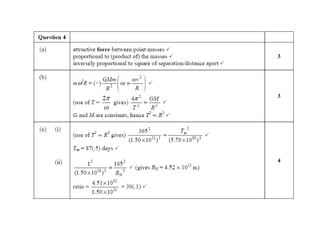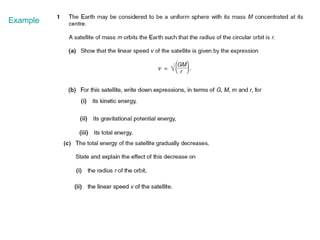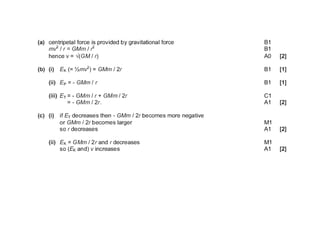1 sur 56

### A2 gravitational field KYUEM Physics

• 1.
• 2.
• 3. Gravity is a very mysterious force. Nobody knows why objects have this attractive force between them, even if they are far apart. This attraction occurs for any object with mass, however small. The force is very small, and always attractive . We never get a repulsive gravitational force.
• 4.
• 5.
• 6.
• 7. Newton found that all objects accelerate towards the Earth at 9.81 m/s 2 . He also worked out the moon has an acceleration towards the Earth of 2.72 m/s 2 . Therefore:   Acceleration of Moon = 2.72 × 10 -3 m/s 2 = 1 = 1 Acceleration of apple 9.81 m/s 2 3610 (60.1) 2 He also worked out the distances between the moon and the centre of the Earth and the distance between the apple and the centre of the Earth. Their ratio also turned out to be 60.1:1.
• 8.
• 9.
• 10. Gravity is a very small force, but it exists between all objects.  There is a tiny gravitational attraction between you and the student sitting next to you in your physics lesson.  You can't feel it because it's negligible.  The only reason we feel gravity is that the Earth is a very large object.  Remember that as well as the Earth attracting us towards it, we are attracting the Earth towards us.  The tiny attraction has important implications for dust particles coming together in space to form stars and planets.
• 11.
• 12.
• 13.
• 14. Gravitational Field Strength We are all familiar with the magnetic field of a bar magnet.  The field lines show up as areas of attraction and repulsion at the poles and the concentration of field lines at any point.  The closer we are to the magnet, the stronger the lines of force.
• 15. We can do a similar exercise with a gravity field; only this time there are attractive forces involved and no repulsive forces.  The Earth, in common with many other planets, is very nearly a perfect sphere (relatively smoother than a billiards ball).  Its gravity field is radial with the pull being directly towards the centre.  The closer in we get, the stronger the pull.
• 16. The concentration of gravitational field lines is an indication of the gravitational field strength at any point, which is formally defined as:   The gravitational force per unit mass at that point .
• 17. So we can write that statement as:   g = gravitational force = F mass m [Units – newtons per kilogram (N/kg)] The gravitational force per unit mass at that point . You will have met the expression F/m in the context of a = F/m, so it doesn’t take a genius to see that gravitational field strength is the same thing as acceleration. A gravitational field strength of 9.81 N/kg causes an acceleration of 9.81 m/s2.
• 18.
• 19.
• 20.
• 22.
• 23.
• 24. If there is always an attraction between masses separated at a certain distance, how can electron be in its orbit without being attracted to the nucleus? Similarly, how can planet orbiting around the sun if there exist a large force that attracts the planet and the sun towards each? Or how can a satellites be orbiting around the Earth?
• 25. The motion of an orbiting satellite can be described by the same motion characteristics as any object in circular motion. The velocity of the satellite would be directed tangent to the circle at every point along its path. The acceleration of the satellite would be directed towards the center of the circle - towards the central body which it is orbiting. And this acceleration is caused by a net force which is directed inwards in the same direction as the acceleration.
• 26. This centripetal force is supplied by gravity - the force which universally acts at a distance between any two objects which have mass. Were it not for this force, the satellite in motion would continue in motion at the same speed and in the same direction. It would follow its inertial, straight-line path. Like any projectile, gravity alone influences the satellite's trajectory such that it always falls below its straight-line, inertial path .
• 27. Observe that the inward net force pushes (or pulls) the satellite (denoted by blue circle) inwards relative to its straight-line path tangent to the circle. As a result, after the first interval of time, the satellite is positioned at position 1 rather than position 1'. In the next interval of time, the same satellite would travel tangent to the circle in the absence of gravity and be at position 2'; but because of the inward force the satellite has moved to position 2 instead. In the next interval of time, the same satellite has moved inward to position 3 instead of tangentially to position 3'. This same reasoning can be continued to explain how the inward force causes the satellite to fall towards the earth without actually falling into it.
• 28.
• 29.
• 30.
• 31.
• 32. 1) Communications satellites orbit the Earth at a height of 36 000 km. How far is this from the centre of the Earth? If such a satellite has a mass of 250 kg, what is the force of attraction on it from the Earth? It is (3.6 x 10 7 m + 6.4 x 10 6 m) = 4.24 x 10 7 m from the centre of the Earth. (Answer should really be given as 4.2 x 10 7 m –significant figures in physical calculations). The force is F = Gm1m2/r 2 = (6.67 x 10 -11 x 6.0 x 10 24 x 250)/ (4.24 x 10 7 ) 2 . This gives an answer of about 56 N, which for information is about less than the weight as a one year old toddler. Example: Newton’s Law of Gravitation Calculation
• 33. 2) What is the force of attraction from the Earth on you? What do we call this force? What is the force of attraction on the Earth from you? You will need to estimate your own mass in kg. If need to convert, 1 stone is 6.4kg (and there are 14 pounds in a stone). Then use F=Gm1m2/r 2 where r is the radius of the Earth. This force is usually called their weight. The force on the Earth from the student is exactly the same as their first answer, but in the opposite direction.
• 34. 3) What is the force of attraction from the Sun on you? How many times smaller is this than the force of attraction from the Earth on you? Again, you will need to use your own mass, and the equation F=Gm 1 m 2 /r 2 , but this time r is the average distance from the Sun to the Earth. This force should be about 1650 times less than their weight, of the order of 0.3-0.5 N. Small, but not negligible.
• 35. 4) The average force of attraction on the Moon from the Sun is 4.4  10 20 N. Taking the distance from the Sun to the Moon to be about the same as that from the Sun to the Earth, what value of mass does this give for the Moon? m 2 = Fr 2 /Gm 1 = (4.4 x 10 20 x (1.5 x 10 11 ) 2 )/(6.67 x 10 -11 x 2.0 x 10 30 ) = 7.4 x 10 22 kg
• 36. 5) Using the mass of the Moon you calculated in question 4, what is the pull of the Earth on the Moon, if the Moon is 380 000 km away? How does this compare with the pull of the Sun on the Moon? F = Gm 1 m 2 /r 2 = (6.67 x 10 -11 x 6.0 x 10 24 x 7.4 x 10 22 )/ (3.8 x 10 8 ) 2 = 2.1 x 10 20 N The average force of attraction on the Moon from the Sun is 4.4  10 20 N.
• 37. 6. What is the force of attraction between two people, one of mass 80 kg and the other 100 kg if they are 0.5m apart? F = Gm 1 m 2 /r 2 F = G x 100 x 80 / 0.5 2 = 2.14 x 10 -6 N. This is a very small force but it does increase as the people get closer together! Actually this example is not accurate because Newton's law really only applies to spherical objects, or at least objects so far apart that they can be effectively considered as spherical.
• 38. 7. What is the force of attraction between the Earth and the Sun? Mass of the Sun = 2 x 10 30 kg, mass of the Earth = 6 x 10 24 kg, distance from the Earth to the Sun = 1.5 x 10 11 m F = Gm1m2/r 2 F = G x 2 x 10 30 x 6 x 10 24 / [1.5 x 10 11 ] 2 = 6.7 x 10 11 N an enormous force!
• 39.
• 40. The satellite turns eastward (like our Earth) along the Equator. It stays above the same point on Earth all the time. To maintain the same rotational period as the Earth, a satellite in geostationary orbit must be 35,787 km(22,237 miles) above the Earth. At this distance, the satellite can view half of the Earth's surface. (Its viewing area is called its &quot;footprint.&quot;) Because the high-altitude satellite appears to remain fixed in one position (it's really orbiting at the same rate as the Earth turns), it requires no tracking to receive its downlink signal. That is why when we turn our home satellite dish to receive the TV signal from a particular geostationary satellite, we don't have to keep jumping up to adjust its position.
• 41.
• 42.
• 43.
• 44.
• 45.
• 46.
• 47.
• 48.
• 50.
• 52.
• 54.
• 56.